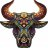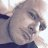# Need help modifying the Chaikin Money Flow Index indicator

W

#### WayneG

##### New member
VIP
I'm modifying the standard Chaikin Money Flow Index according to it interpretation rules. The area betwen -0.05 to 0.05, the gray area, is considered to be a no mans land because of false signals. In the code I've written, see below, I am having trouble getting the red not to fill the gray to +0.5. I've tried several variation from different example, but I'm just no catching the hank of it.Code:

Code:
``````declare lower;
input length = 21;
input upperLine = 1;
input lowerLine = -1;
input pgrayline = 0.05;
input ngrayline = -0.05;
def tmp_var =
if high == low then
volume
else
(close - low - (high - close)) / (high - low) * volume
;

def sum_close = Sum(tmp_var, length);
def total = Sum(volume, length);

plot CMF =
if total == 0 then
0
else
sum_close / total
;
CMF.SetDefaultColor(Color.BLACK);

plot ThirtyLine = .30;
ThirtyLine.SetDefaultColor(GetColor(5));

plot TwentyLine = -.20;
TwentyLine.SetDefaultColor(GetColor(5));

plot ngray = ngrayline;
ngray.SetDefaultColor(Color.GRAY);

plot pgray = pgrayline;
pgray.SetDefaultColor(Color.GRAY);

plot ZeroLine = 0;
ZeroLine.SetDefaultColor(GetColor(5));

def BullPrice = ChaikinMoneyFlow(21);

def BearPrice = -chaikinMoneyFlow(21);
#
#THE NEXT TO LINES OF CODE ARE PROBLEM
#
#
#
#

AddLabel( 1, "Short < -0.05 Long > 0.05 Gray area Choppy", Color.BLACK);

#end code``````
Thanks for you help,
Wayne#### horserider

##### Well-known member
VIP
Messy but may do.

Code:
``````plot ngray = .05;
ngray.SetDefaultColor(Color.GRAY);

plot pgray = -.05;
pgray.SetDefaultColor(Color.GRAY);

#
#THE NEXT TO LINES OF CODE ARE PROBLEM
#
#
#

AddLabel( 1, "Short < -0.05 Long > 0.05 Gray area Choppy", Color.BLACK);

#end code``````

D

#### DeusMecanicus

##### Member
Try this:

Code:
``````#THE NEXT TO LINES OF CODE ARE PROBLEM

plot uppercmf = if cmf > .05 then cmf else double.nan;
uppercmf.hide();
plot lowercmf = if cmf < -.05 then cmf else double.nan;
lowercmf.hide();
#
#
#

AddLabel( 1, "Short < -0.05 Long > 0.05 Gray area Choppy", Color.BLACK);

#end code``````
You lose some shading on the edges when it dips above/below the .05/-.05 levels.Need help with array variables Questions 1Need help paint horizontal pivot lines Questions 5